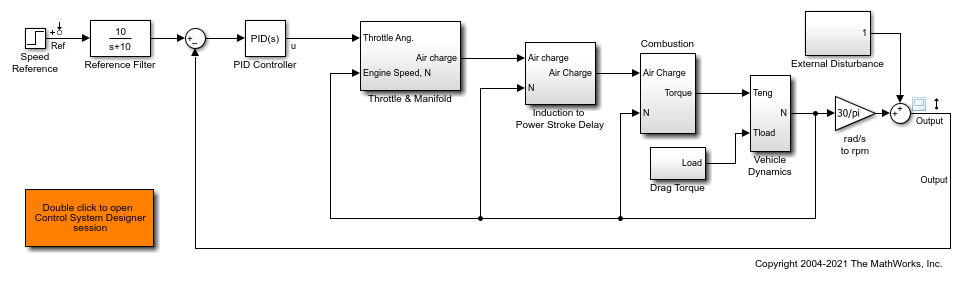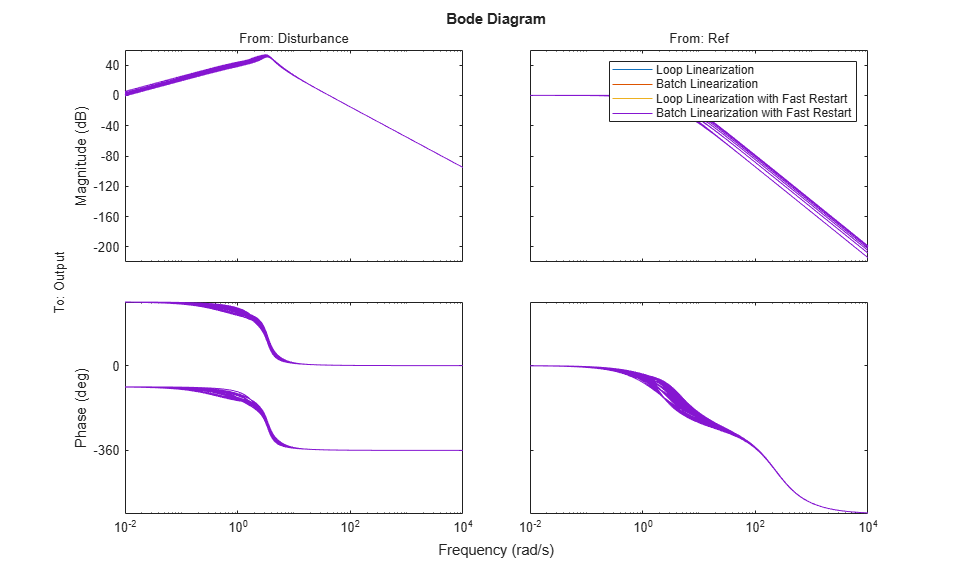Documentation

## Improve Linear Analysis Performance

This example shows how to use the `fastRestartForLinearAnalysis` command to speed up multiple calls to compiling functions in Simulink Control Design such as `findop` and `linearize`.

### Run Linear Analysis Commands in a Loop

In this example, you will trim and linearize a closed loop engine speed control model. The PI control parameters are varied to observe how the closed loop behavior changes at steady state. Since `linearize` and `findop` are called in a loop, the model will be compiled 2*N + 1 times including the first call to `operspec`.

Open the engine speed control model and obtain the linear analysis points from the model.

```mdl = 'scdspeedctrl'; open_system(mdl); io = getlinio(mdl); fopt = findopOptions('DisplayReport','off'); ```Configure the PI controller to use the base workspace variables `kp` and `ki`.

```blk = [mdl,'/PID Controller']; set_param(blk,'P','kp'); set_param(blk,'I','ki'); ```

Create a grid of parameters to vary.

```vp = 0.0005:0.0005:0.003; vi = 0.0025:0.0005:0.005; [KP,KI] = ndgrid(vp,vi); N = numel(KP); sz = size(KP); ```

Initialize the base workspace variables.

```kp = KP(1); ki = KI(1); ```

Run the loop and record execution time.

```t = cputime; ops = operspec(mdl); for i = N:-1:1 kp = KP(i); ki = KI(i); % trim the model op = findop(mdl,ops,fopt); [j,k] = ind2sub(sz,i); % linearize the model sysLoop(:,:,j,k) = linearize(mdl,io,op); end ```

Calculate the elapsed time.

```timeElapsedLoop = cputime - t; ```

### Run Linear Analysis Commands in Batch

Rather than loop over the parameters, `findop` and `linearize` can accept a batch parameter variation structure directly to reduce the number of times the model is compiled. The model will be compiled 3 times with calls to `operspec`, `findop`, and `linearize`.

Run and record execution time.

```t = cputime; ops = operspec(mdl); ```

Create the batch parameter structure.

```params(1).Name = 'kp'; params(1).Value = KP ; params(2).Name = 'ki'; params(2).Value = KI ; ```

Trim the model across the parameter set.

```op = findop(mdl,ops,params,fopt); ```

Linearize the model across the parameter and operating point set.

```sysBatch = linearize(mdl,io,op,params); ```

Calculate the elapsed time.

```timeElapsedBatch = cputime - t; ```

### Run Linear Analysis Commands in a Loop with fastRestartForLinearAnalysis

The `fastRestartForLinearAnalysis` command will configure the model to minimize compilations even when compiling commands are run inside a loop. The model will be compiled once with calls to `operspec`, `findop`, and `linearize` in a loop.

Run the loop and record execution time with `fastRestartForLinearAnalysis` "on".

```t = cputime; ```

Turn `fastRestartForLinearAnalysis` "on". Provide AnalysisPoints to minimize compilations between calls to `findop` and `linearize`.

```fastRestartForLinearAnalysis(mdl,'on','AnalysisPoints',io); ops = operspec(mdl); for i = N:-1:1 kp = KP(i); ki = KI(i); % make sure the block initialization is called after the parameters % are updated when the model is in a compiled state Simulink.Block.eval(blk); % trim the model op = findop(mdl,ops,fopt); [j,k] = ind2sub(sz,i); % linearize the model sysFastRestartLoop(:,:,j,k) = linearize(mdl,io,op); end ```

Turn `fastRestartForLinearAnalysis` "off". This will uncompile the model.

```fastRestartForLinearAnalysis(mdl,'off'); ```

Calculate the elapsed time.

```timeElapsedFastRestartLoop = cputime - t; ```

### Run Linear Analysis Commands in Batch with fastRestartForLinearAnalysis

Performance can be further improved by turning `fastRestartForLinearAnalysis` "on" and executing the batch `linearize` and `findop` commands. The model will be compiled once with calls to `operspec`, `findop`, and `linearize`.

Run and record execution time with fast restart for linear analysis on.

```t = cputime; ```

Turn `fastRestartForLinearAnalysis` "on". Provide AnalysisPoints to minimize compilations between the calls to `findop` and `linearize`.

```fastRestartForLinearAnalysis(mdl,'on','AnalysisPoints',io); ops = operspec(mdl); ```

Create the batch parameter structure.

```params(1).Name = 'kp'; params(1).Value = KP ; params(2).Name = 'ki'; params(2).Value = KI ; ```

Trim the model across the parameter set.

```op = findop(mdl,ops,params,fopt); ```

Linearize the model across the parameter and operating point set.

```sysFastRestartBatch = linearize(mdl,io,op,params); ```

Turn `fastRestartForLinearAnalysis` "off". This will uncompile the model.

```fastRestartForLinearAnalysis(mdl,'off'); ```

Calculate the elapsed time.

```timeElapsedFastRestartBatch = cputime - t; ```

### Comparing the Results

Compare the linearization results of the 4 methods. The bode plot below shows each method returns the same results.

Specify the indices to compare.

```compareIdx = 1:N; bode(... sysLoop (:,:,compareIdx),... sysBatch (:,:,compareIdx),... sysFastRestartLoop (:,:,compareIdx),... sysFastRestartBatch(:,:,compareIdx)); legend(... 'Loop Linearization' ,... 'Batch Linearization' ,... 'Loop Linearization with Fast Restart',... 'Batch Linearization with Fast Restart') ```Compile the elapsed time and speed-up ratio for each method in a table. Significant performance gains can be achieved by using batch trimming/linearization as well as `fastRestartForLinearAnalysis`.

```Method = ["Loop","Batch","Fast Restart Loop","Fast Restart Batch"]'; TimeElapsed = [timeElapsedLoop,timeElapsedBatch,timeElapsedFastRestartLoop,timeElapsedFastRestartBatch]'; SpeedUpFactor = TimeElapsed(1)./TimeElapsed; TimeElapsedTable = table(Method,TimeElapsed,SpeedUpFactor) ```
```TimeElapsedTable = 4x3 table Method TimeElapsed SpeedUpFactor ____________________ ___________ _____________ "Loop" 89.31 1 "Batch" 35.11 2.5437 "Fast Restart Loop" 36.74 2.4309 "Fast Restart Batch" 36.59 2.4408 ```

```bdclose(mdl); ```